§5  多项式

[整值多项式]  当变数x为整数时,一多项式f(x)的值常为整数，则这种多项式称为整值多项式。

整系数多项式是整值多项式一特例。

整值多项式表达式：

1°  n次整值多项式必可表为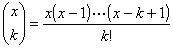2°  整值奇多项式(满足f(x)=f(x))必可表为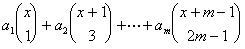3°  整值偶多项式(满足f(x)=f(x)) 必可表为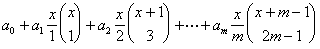[可约多项式与不可约多项式]  f(x)为一有理系数多项式,若有非常数的有理系数多项式g(x)h(x),使得

f(x)=g(x)h(x)

[高斯定理]  f(x)为一整系数多项式,在有理数域上可约,则必有二整系数多项式g(x)h(x),使得

f(x)=g(x)h(x)

[爱森斯坦判别法]

1°为一整系数多项式.若有素数p,使得f(x)为不可约多项式.

2°2n+1次整系数多项式,若有素数p,使得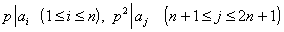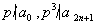f(x)为不可约多项式.

[派朗判别法]

1°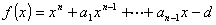(i)(ii)(iii)实数)

f(x)为不可约多项式.

2°

``

(i)(ii)f(x)为不可约多项式.

3°``

f(x)为不可约多项式.

4°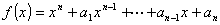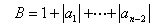f(x)为不可约多项式.

5°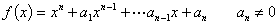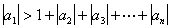f(x)为不可约多项式.

[多项式的整除性]  f(x)g(x)为二有理系数多项式,g(x)不恒为零,若有一多项式h(x),使得

f(x)=g(x)h(x)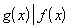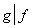以下°f表示多项式f(x)的次数.

多项式的整除性具有下列性质:

1°2°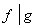,fg仅相差一常数因子.

3°,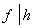4°,°f°g,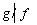,f称为g的真因式,显然°f<°g.

5°  p(x)为一不可约多项式,,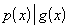.

6°  p(x)为一不可约多项式,

f(x)=0,p(x)=0

[多项式的带余除法]  f(x),g(x)为任意多项式,g(x)不恒为零,则必有两个多项式q(x)r(x),使得

f(x)=g(x)q(x)+r(x)

[多项式的辗转相除法]  多项式的辗转相除法与整数的辗转相除法的定义,公式完全类似,只须把本章§1(1)式中的文字符号看作多项式就行了.

同样,多项式的唯一分解定理,最高公因式和最低公倍式,多项式互素等概念和公式与整数一节完全类似,只须把相应公式中的符号看作多项式就行了.

求多项式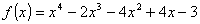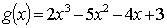为了避免分数,先用2f(x),然后再用g(x)去除2f(x):``

在计算过程中,2乘第一个差,因而商式变了样,但余式只获得一个数因子2,这不关紧要.3g(x),除以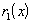: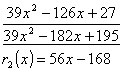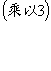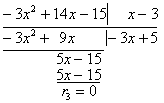[同余式]

1°  多项式模同余式  m(x)为一多项式,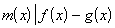2°  素数模同余式  p为素数,f(x)g(x)为整系数多项式,若各项对应系数都对模p同余,则称此二多项式对模p同余,记作3°  重模同余式  p为素数,(x)为多项式,f(x)g(x)(x)的倍式,mod p,则称f(x)g(x)对重模p,(x)同余,记作

f(x)g(x)[费马定理的推广]  p为素数,(x)n次不可约多项式,mod p,则对任一非(x)的倍式的多项式f(x),mod p,恒有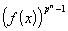1f(x)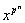x